# Thread: Derivative Problem (x^2)^(e^x)

1. ## Derivative Problem (x^2)^(e^x)

How do you find the derivative of (x^2)^(e^x)

I get stuck after applying the chain rule and I get (2x * e^x(x^2)^(e^x)-1)

Is this correct ?

Please help

Cheers

2. The standard method is to take natural logs of both sides and then differentiate...

However, - Wolfram|Alpha does it with the chain rule for two inner functions...

$\displaystyle \frac{d}{dx}\ f(u(x), v(x)) = \frac{\partial f}{\partial u} \frac{du}{dx} + \frac{\partial f}{\partial v} \frac{dv}{dx}$

... which doesn't mean we have to, of course, but I fancy this picture

makes it palatable...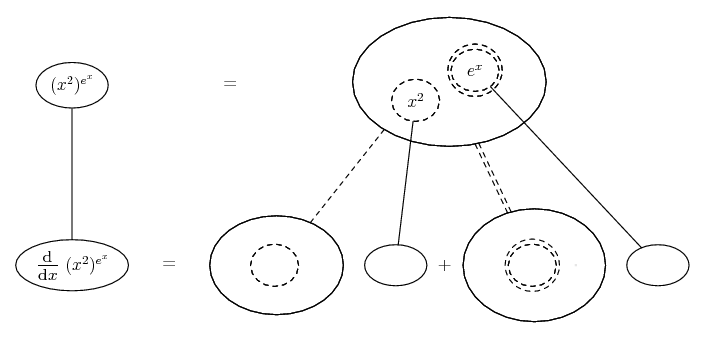... where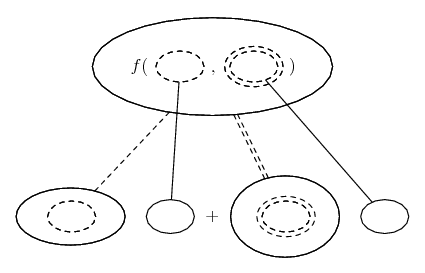... is the double version of...... the ordinary chain rule. As with that, straight continuous lines differentiate downwards (integrate up) with respect to x, and the straight dashed lines similarly but with respect to the (corresponding) dashed balloon expression which is (one of) the inner function(s) of the composite expression. So...

Spoiler: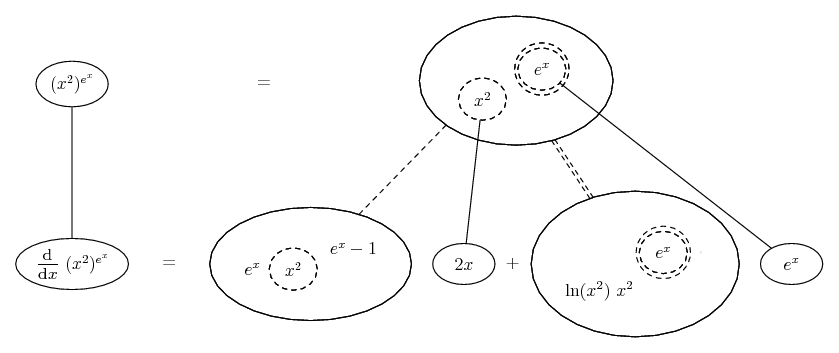You do, of course, need logarithmic differentiation to see the logic of the double-dashed differentiation, i.e. the logic of...
$\displaystyle \frac{d}{du}\ a^u = \ln a\ a^u$

_____________________________________
Don't integrate - balloontegrate!

Balloon Calculus: Standard Integrals, Derivatives and Methods

Balloon Calculus Drawing with LaTeX and Asymptote!

3. By the way, just in case a picture helps (and pardon if not) with the 'logarithmic' way also, we can solve the bottom row for dy/dx in...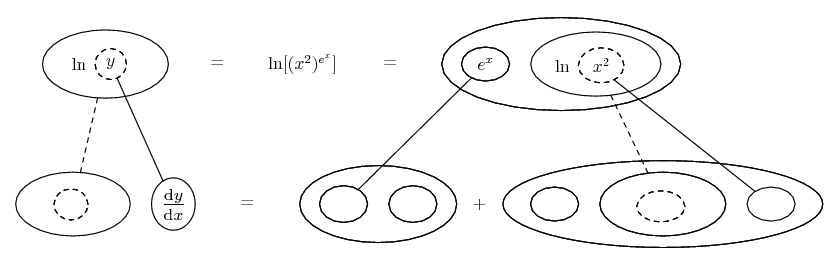... where the chain rule (see previous) is wrapped in the legs-uncrossed version of...... the product rule, in which straight continuous lines still differentiate downwards with respect to x.

Spoiler: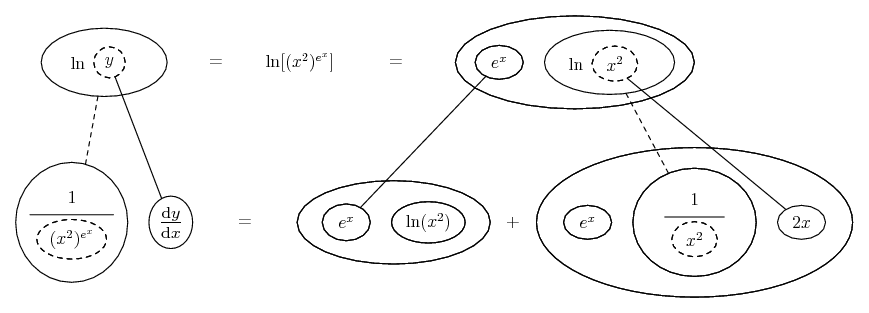...which will, of course, agree with the previous result.

_________________________________________
Don't integrate - balloontegrate!

Balloon Calculus; standard integrals, derivatives and methods

Balloon Calculus Drawing with LaTeX and Asymptote!

4. In case you want a more traditional method than given above:

$\displaystyle f(x) = \left( x^2 \right) ^ {e^x}$

First we want to rewrite the function to move the $\displaystyle e^x$ term out of the exponent. To do this, we must take the $\displaystyle \ln$ of both sides.

$\displaystyle \implies \ln f(x) = \ln \left[ \left( x^2 \right) ^ {e^x} \right]$

$\displaystyle \implies \ln f(x) = e^x \ln x^2$

Now take a derivative:

$\displaystyle \implies \frac{d}{dx} \ln f(x) = \frac{d}{dx} \left[ e^x \ln x^2 \right]$

$\displaystyle \implies \frac{f'(x)}{f(x)} = \frac{d}{dx} \left[ e^x \ln x^2 \right]$

$\displaystyle \implies f'(x) = f(x) \cdot \frac{d}{dx} \left[ e^x \ln x^2 \right]$

$\displaystyle \implies f'(x) = \left( x^2 \right) ^ {e^x} \cdot \frac{d}{dx} \left[ e^x \ln x^2 \right]$

I'll let you finish with the calculation.

#### Search Tags

derivative, problem, x2ex